# KG Support & Resistance indicator - update help34

I have been trying to get this indicator working with no luck. It attaches to the chart and only adds little red dots the all the candles. I want to know how to display the label [M15 Resistence] [M15 Support]\ [H1Resistence] [H1 Support]\ [H4 Resistence] [H4Support]\[D1 Resistence] [D1Support] on my chart as the pic showed?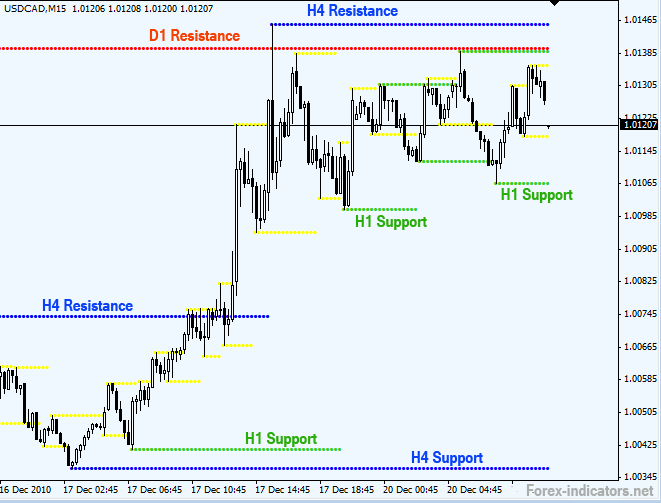How to change the line's defalt width?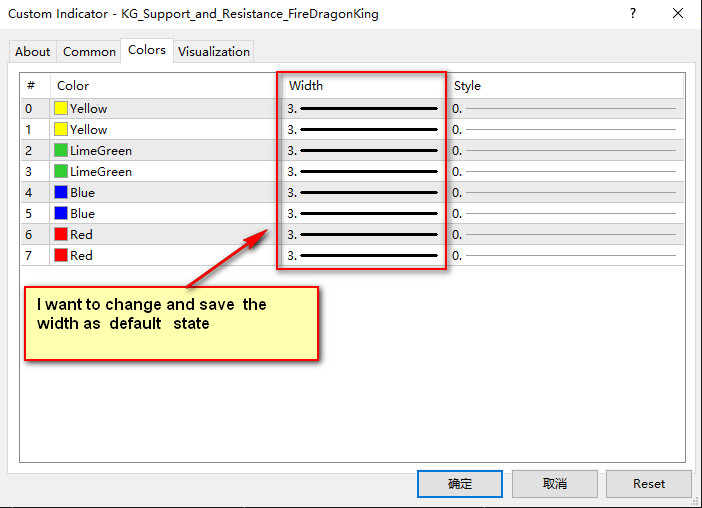I would like to try it out on my personal trading system I have been developing. I know nothing about code. I am pretty much looking at a foreign language. If anyone out there would be willing to take a look and possibly update the code for me , that would be great. Please let me know. Thanks .

```//+------------------------------------------------------------------+
//|                                      KG Support & Resistance.mq4 |
//|                      Copyright ?2007, MetaQuotes Software Corp. |
//|                                        http://www.metaquotes.net |
//+------------------------------------------------------------------+

#property indicator_chart_window
#property indicator_buffers 8
#property indicator_color1 Yellow
#property indicator_color2 Yellow
#property indicator_color3 LimeGreen
#property indicator_color4 LimeGreen
#property indicator_color5 Blue
#property indicator_color6 Blue
#property indicator_color7 Red
#property indicator_color8 Red
//---- input parameters

//---- buffers
double ExtMapBuffer1[];
double ExtMapBuffer2[];
double ExtMapBuffer3[];
double ExtMapBuffer4[];
double ExtMapBuffer5[];
double ExtMapBuffer6[];
double ExtMapBuffer7[];
double ExtMapBuffer8[];
int KG;
//+------------------------------------------------------------------+
//| Custom indicator initialization function                         |
//+------------------------------------------------------------------+
int init()
{
//---- indicators
SetIndexStyle(0,DRAW_ARROW);
SetIndexDrawBegin(0,KG-1);
SetIndexBuffer(0,ExtMapBuffer1);
SetIndexLabel(0,"Resistance M15");
SetIndexArrow(0, 158);
SetIndexStyle(1,DRAW_ARROW);
SetIndexDrawBegin(1,KG-1);
SetIndexBuffer(1,ExtMapBuffer2);
SetIndexLabel(1,"Support M15");
SetIndexArrow(1, 158);
SetIndexStyle(2,DRAW_ARROW);
SetIndexDrawBegin(2,KG-1);
SetIndexBuffer(2,ExtMapBuffer3);
SetIndexLabel(2,"Resistance H1");
SetIndexArrow(2, 158);
SetIndexStyle(3,DRAW_ARROW);
SetIndexDrawBegin(3,KG-1);
SetIndexBuffer(3,ExtMapBuffer4);
SetIndexLabel(3,"Support H1");
SetIndexArrow(3, 158);
SetIndexStyle(4,DRAW_ARROW);
SetIndexDrawBegin(4,KG-1);
SetIndexBuffer(4,ExtMapBuffer5);
SetIndexLabel(4,"Resistance H4");
SetIndexArrow(4, 158);
SetIndexStyle(5,DRAW_ARROW);
SetIndexDrawBegin(5,KG-1);
SetIndexBuffer(5,ExtMapBuffer6);
SetIndexLabel(5,"Support H4");
SetIndexArrow(5, 158);
SetIndexStyle(6,DRAW_ARROW);
SetIndexDrawBegin(6,KG-1);
SetIndexBuffer(6,ExtMapBuffer7);
SetIndexLabel(6,"Resistance D1");
SetIndexArrow(6, 158);
SetIndexStyle(7,DRAW_ARROW);
SetIndexDrawBegin(7,KG-1);
SetIndexBuffer(7,ExtMapBuffer8);
SetIndexLabel(7,"Support D1");
SetIndexArrow(7, 158);
//----
return(0);
}
//+------------------------------------------------------------------+
//| Custor indicator deinitialization function                       |
//+------------------------------------------------------------------+
int deinit()
{
return(0);
}
//------------------------------------------------------------------
bool Fractal (string M,int P, int shift)
{
if (Period()>P) return(false);
P=P/Period()*2+MathCeil(P/Period()/2);
if (shift<P)return(false);
if (shift>Bars-P)return(false);
for (int i=1;i<=P;i++)
{
if (M=="U")
{
if (High[shift+i]>High[shift])return(false);
if (High[shift-i]>=High[shift])return(false);
}
if (M=="L")
{
if (Low[shift+i]<Low[shift])return(false);
if (Low[shift-i]<=Low[shift])return(false);
}
}
return(true);
}
//------------------------------------------------------------------
int start()
{
int D1=1440, H4=240, H1=60, M15=15;
KG=Bars-1440;
while(KG>=0)
{
if (Fractal("U",M15,KG)==true) ExtMapBuffer1[KG]=High[KG];
else ExtMapBuffer1[KG]=ExtMapBuffer1[KG+1];
if (Fractal("L",M15,KG)==true) ExtMapBuffer2[KG]=Low[KG];
else ExtMapBuffer2[KG]=ExtMapBuffer2[KG+1];
if (Fractal("U",H1,KG)==true) ExtMapBuffer3[KG]=High[KG];
else ExtMapBuffer3[KG]=ExtMapBuffer3[KG+1];
if (Fractal("L",H1,KG)==true) ExtMapBuffer4[KG]=Low[KG];
else ExtMapBuffer4[KG]=ExtMapBuffer4[KG+1];
if (Fractal("U",H4,KG)==true) ExtMapBuffer5[KG]=High[KG];
else ExtMapBuffer5[KG]=ExtMapBuffer5[KG+1];
if (Fractal("L",H4,KG)==true) ExtMapBuffer6[KG]=Low[KG];
else ExtMapBuffer6[KG]=ExtMapBuffer6[KG+1];
if (Fractal("U",D1,KG)==true) ExtMapBuffer7[KG]=High[KG];
else ExtMapBuffer7[KG]=ExtMapBuffer7[KG+1];
if (Fractal("L",D1,KG)==true) ExtMapBuffer8[KG]=Low[KG];
else ExtMapBuffer8[KG]=ExtMapBuffer8[KG+1];
KG--;
}

return(0);
}
//+------------------------------------------------------------------+```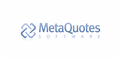MetaQuotes Software Corp.
• www.metaquotes.net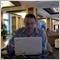115863

When you post code please use the CODE button (Alt-S)!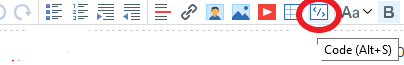115863

.. and if no one helps on this thread so you can go to the Freelance service for example - https://www.mql5.com/zh/job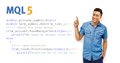• www.mql5.com
MQL5应用程序开发者最大的自由职业者服务版块34

Sergey Golubev:

Sergey Golubev:

Thank you for reminding me, I know.